# Complex Fourier Series into a Cosine Series

## Homework Statement

a. Represent f(x)=|x| in -2<x<2 with a complex Fourier series

b. Show that the complex Fourier Series can be rearranged into a cosine series

c. Take the derivative of that cosine series. What function does the resulting series represent?
[/B]

## Homework Equations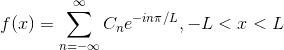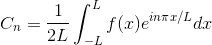[/B]

## The Attempt at a Solution

[/B]
Ok, so the first part seems pretty straight forward, I plugged the variables in, and due to the absolute value broke the integral up from -2 to 0 and then from 0 to -2 and added them together.

Providing I made no errors (I hope...), I get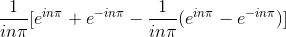Using Euler's, I simplify this to: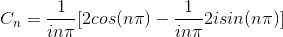which, since for each n the sin (n pi) will become 0, my assumption is the above simply becomes (...?):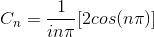I am completely befuddled as to how to move into part b though... I also don’t know what to do with the n’s of the summation. So far, all the cosine series I've done so far have been summed from 0 to ∞ - I don’t know what to do with the -∞ to 0 part of the summation.

Any help would be greatly appreciated. Thanks for looking...

Last edited by a moderator:

blue_leaf77
Homework Helper
I suggest that you check again your calculation of ##C_n##. The integral of ##C_n## can be simplified to
$$C_n = \frac{1}{2L} \int_{-L}^L f(x) e^{in\pi x/L} dx = \frac{1}{2L} \int_{-L}^L f(x) \cos\frac{n\pi x}{L} dx$$
The integral with ##i\sin\frac{n\pi x}{L}## vanishes because the product ##f(x)\sin\frac{n\pi x}{L}## forms an odd function. In this regard, I would expect ##C_n## to be real for any ##n##.

BvU
Homework Helper
I don’t know what to do with the -∞ to 0 part of the summation.
Note that a summation index is just a place holder that takes on a value: $$\sum_{i=-\infty}^0 g(i) = \sum_{i=0}^{+\infty} g(-i)$$

 should have used ##n## instead of ##i## to avoid confusion, sorry. I mean a sum over integer numbers

Thank you two so much for your insight! I was making a silly error when integrating the absolute value.

I'm still getting a little stuck (very stuck) on b: "Show that the complex Fourier Series can be rearranged into a cosine series"

So, for part A: Represent /x/ with a complex Fourier Series, after correcting my C_n calculations, I got the answer of:

$C_n = \frac{2 cos (n\pi ) -2}{(n\pi)^2}$

$f(x) = \sum_{-\infty}^{\infty} C_ne^{-in\pi x /2}$ , -2 < x < 2

Now, for part B, I have to rearrange this into a cosine series....I was trying to start by using Euler's identity to start:

$f(x) = \sum_{-\infty}^{\infty} C_ne^{-in\pi x /2}$

$= C_n [cos (\frac{n\pi x}{2})-isin(\frac{n\pi x}{2})]$

....and this is as far as I get. I think (if my understanding of the material is correct) that I could make a conceptual argument as to why it can be just represented with the cosine series using some graphs that have been shown to me, but I don't know what I need to do to show it via formulas....

blue_leaf77
Homework Helper
....and this is as far as I get. I think (if my understanding of the material is correct) that I could make a conceptual argument as to why it can be just represented with the cosine series using some graphs that have been shown to me, but I don't know what I need to do to show it via formulas....
Except for ##n=0##, for each ##n=m##, you also have ##n=-m## in the Fourier series. You have figured out that the Fourier coefficient looks like
$$C_n = \frac{2 \cos (n\pi ) -2}{(n\pi)^2}$$
Now compare this coefficient for ##n=m## and ##n=-m##, how do you conclude about the relation between them? Then consider the two terms out of the series which correspond to ##n=\pm m##
$$C_m e^{-im\pi x/L} + C_{-m} e^{im\pi x/L}$$
Taking the relation between ##C_m## and ##C_{-m}## you have found before, how can you simplify them?

BvU
Homework Helper
Very good you fixed your intergration issue. ##1\over n^2## sounds a lot better than ##1\over n##.
You are way overthinking things: part a asked for the complex coefficients ##C_n## in $$f(x) = \sum_{n = -\infty}^\infty C_n e^{-in\pi/L}$$ and you found them.

Drat, blueleaf was faster (a lot faster!) and he's good.

blue_leaf77
Homework Helper
Drat, blueleaf was faster (a lot faster!) and he's good.
May be, the timezone also has effect? I live in GMT+07.00 where it's currently approaching the dusk, if you are in the same zone as the US then I imagine it must be around breakfast time right now.

BvU
Homework Helper
GMT+1 -- after lunch dipEven the GMT+1 isn't good enough/unambiguous nowadays. They cheat and call it CET

So I casually stumbled upon this problem and tried solving the problem on my own just for fun, but doesn't quite get the answer to part B and C. For B, do you just ignored the sine portion of the Euler's identity since f(x) is an even function?

Last edited:
blue_leaf77
Homework Helper
For B, do you just ignored the sine portion of the Euler's identity since f(x) is an even function?
If you read through this post at least until post #6, you should get the hint why the sine terms do not appear.

Except for ##n=0##, for each ##n=m##, you also have ##n=-m## in the Fourier series. You have figured out that the Fourier coefficient looks like
$$C_n = \frac{2 \cos (n\pi ) -2}{(n\pi)^2}$$
Now compare this coefficient for ##n=m## and ##n=-m##, how do you conclude about the relation between them? Then consider the two terms out of the series which correspond to ##n=\pm m##
$$C_m e^{-im\pi x/L} + C_{-m} e^{im\pi x/L}$$
Taking the relation between ##C_m## and ##C_{-m}## you have found before, how can you simplify them?

Could you elaborate a bit on how this is supposed to rearrange the complex series to cosine series? Sorry, just really lost haha

blue_leaf77
Homework Helper
Take a look at this.

Take a look at this.

So this is the work i've done and attempt to solve the last part. Could you take a look and see if there are mistakes? Thanks a lot.

BvU
Homework Helper
Well, it doesn't represent the sine function...
beware the PF rules: the OP has to have something to do left over ...

Thanks for all your help, all. The posts through #6 clued me in as to how to actually show the sines do indeed disappear, and now I understand WHY, instead of just taking it as gospel that it does. The work shown above helped me confirm my understanding (and the work I turned in) was correct. And, lastly, that derivative represents f'(x) = d/dx /x/.

Really, really appreciate it! I wasn't grasping some of the concepts at play here; thanks so much!

vela
Staff Emeritus